# Colleges with the highest SAT scores in Massachusetts

Top 10 colleges in Massachusetts with the highest SAT scores
Looking for the colleges with the highest SAT scores in Massachusetts? Well you're in luck! We've compiled a national college database and have created a list of the top 10 universities with the highest SAT scores in Massachusetts. These are the schools whose applicants had the highest average SAT scores in Massachusetts. And, since these tests are meant to determine academic prowess, they are arguably the schools with the most academically proficient students. You could even say these are the best colleges in Massachusetts. We also include each college's ACT scores and acceptance rate so that you can see where you would have the easiest or hardest time getting in. Read on to find out more.

## Massachusetts Institute of Technology SAT scores

The average SAT score for Massachusetts Institute of Technology is 1535.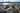The average SAT score of 1535 breaks down into:

• SAT math: 790

The average ACT score for Massachusetts Institute of Technology is 35 and their acceptance rate is 6.7%.

## Harvard University SAT scores

The average SAT score for Harvard University is 1520.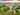The average SAT score of 1520 breaks down into:

• SAT math: 770

The average ACT score for Harvard University is 34 and their acceptance rate is 4.7%.

## Franklin W. Olin College of Engineering SAT scores

The average SAT score for Franklin W. Olin College of Engineering is 1501.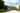The average SAT score of 1501 breaks down into:

• SAT math: 770

The average ACT score for Franklin W. Olin College of Engineering is 34 and their acceptance rate is 15.7%.

## Amherst College SAT scores

The average SAT score for Amherst College is 1480.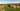The average SAT score of 1480 breaks down into:

• SAT math: 755

The average ACT score for Amherst College is 33 and their acceptance rate is 11.3%.

## Williams College SAT scores

The average SAT score for Williams College is 1479.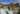The average SAT score of 1479 breaks down into:

• SAT math: 750

The average ACT score for Williams College is 33 and their acceptance rate is 13%.

## Northeastern University SAT scores

The average SAT score for Northeastern University is 1465.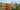The average SAT score of 1465 breaks down into:

• SAT math: 750

The average ACT score for Northeastern University is 34 and their acceptance rate is 18.1%.

## Tufts University SAT scores

The average SAT score for Tufts University is 1465.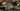The average SAT score of 1465 breaks down into:

• SAT math: 750

The average ACT score for Tufts University is 33 and their acceptance rate is 15%.

## Smith College SAT scores

The average SAT score for Smith College is 1430.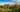The average SAT score of 1430 breaks down into:

• SAT math: 720

The average ACT score for Smith College is 32 and their acceptance rate is 31%.

## Wellesley College SAT scores

The average SAT score for Wellesley College is 1425.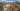The average SAT score of 1425 breaks down into:

• SAT math: 720

The average ACT score for Wellesley College is 32 and their acceptance rate is 19.5%.

## Boston College SAT scores

The average SAT score for Boston College is 1420.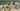The average SAT score of 1420 breaks down into:

• SAT math: 725